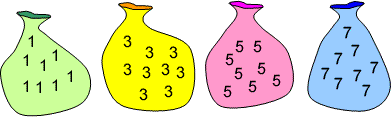#### You may also like### Consecutive Numbers

An investigation involving adding and subtracting sets of consecutive numbers. Lots to find out, lots to explore.### Roll These Dice

Roll two red dice and a green dice. Add the two numbers on the red dice and take away the number on the green. What are all the different possible answers?### Domino Square

Use the 'double-3 down' dominoes to make a square so that each side has eight dots.

# Make 37

## Make 37

Four bags contain a large number of $1$s, $3$s, $5$s and $7$s.Pick any ten numbers from the bags above so that their total is $37$.

### Why do this problem?

Make 37 offers learners the chance to apply basic number properties and operations, and will challenge the idea of mathematical problems having one right answer. It is an opportunity to encourage children to generalise and, even at this level, they can be asked to prove these generalisations.

### Possible approach

One way to start is to ask pupils to make $16$ using any four of the numbers. Make sure they have paper or mini whiteboards for working on. They will quickly find a variety of answers.

Declare this is too easy and set them on the task of making $37$ using ten of the numbers. Then give the pupils time to "play", trying out different numbers for themselves. It would be a good idea to encourage them to check their results with a neighbour! Refrain from asking if it is possible; wait until a pupil suggests it can't be done, to which the response could be: "Why can't it be done?"

It might be appropriate to ask pupils what totals they have been able to make and what they notice about these totals. Turning pupils' attention to the properties of the totals that are possible will encourage them to suggest conclusions. Have they noticed that all the totals are even? This may alert them to the fact that making $37$ is much harder than they thought or does not seem to be possible.

Having given the group more time to work in pairs or small groups, draw their ideas together using the key questions below. Once they have drawn conclusions based on odd and even numbers, challenge them to convince you this is the case. Drawing diagrams of odd and even numbers may help pupils to visualise the mathematics.

A follow-up question along the lines of "How could you change the problem so that we can make $37$ from ten numbers?" or "How can we change the problem so that it is possible?" provides a useful opportunity to gauge how fully the children have understood the ideas.

### Key questions

What do you notice about the numbers in the bags? What do they have in common?
What totals have you managed to make using ten numbers? Do they have anything in common?
Can you make $37$ with a different number of numbers? How many?
Can you explain why?

### Possible extension

This problem could be used as a starter before tackling What Numbers Can We Make? and the more challenging Take Three from Five

### Possible support

You can use a lower total and fewer numbers to make the arithmetic easier without losing the mathematical thinking and reasoning. For example, the challenge could be to make $15$ using four numbers.